# Area & Perimeter of Compound Shapes

In this section we look at ways of finding the area of shapes which are made up from other shapes.

## Exercises

Work out the answers to the questions below and fill in the boxes. Click on the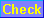button to find out whether you have answered correctly. If you are right then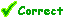will appear and you should move on to the next question. If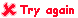appears then your answer is wrong. Click onto clear your original answer and have another go. If you can't work out the right answer then click on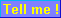to see the answer.

Question 1
Find the area of these shapes. One square on the grid is 1 cm˛.

(a)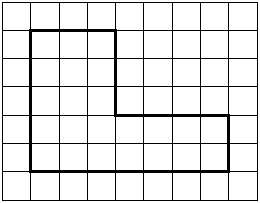cm˛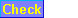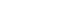(b)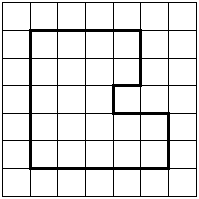cm˛Question 2
Find the areas and perimeters of the shapes below.
The diagrams have not been drawn accurately, so you may find it helpful to sketch them on paper.

 (a)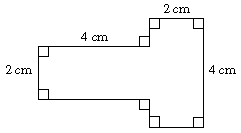Area = cm˛Perimeter = cm(b)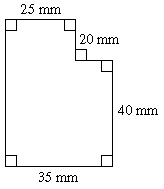Area = mm˛Perimeter = mm(c)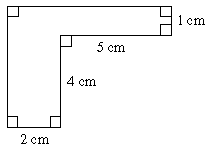Area = cm˛Perimeter = cm(d)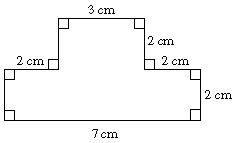Area = cm˛Perimeter = cm(e)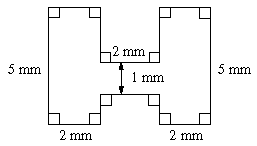Area = mm˛Perimeter = mm(f)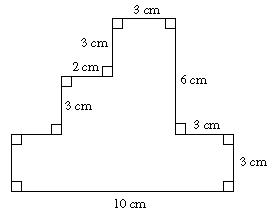Area = cm˛Perimeter = cmQuestion 3
Work out the shaded area in each of the diagrams below.
HINT: You may find it easiest to work out the area of the unshaded part and subtract it from the area of the outer rectangle.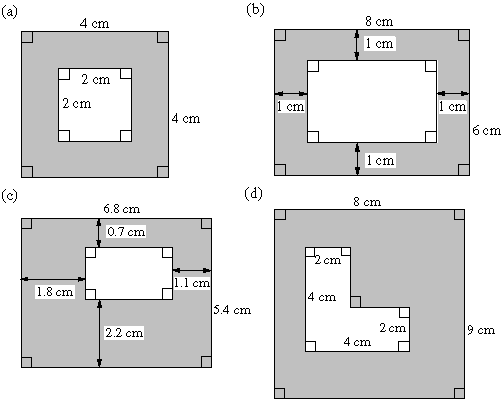(d) cm˛Question 4
Find the areas and perimeters of the shapes below.
The diagrams have not been drawn accurately, so you may find it helpful to sketch them on paper.

 (a)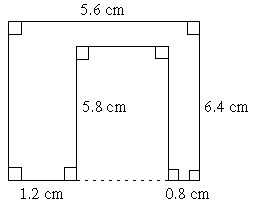Area = cm˛Perimeter = cm(b)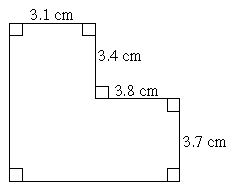Area = cm˛Perimeter = cmYour overall score for this section is Correct Answers You answered questions correctly out of the questions in this section. Incorrect Answers There were questions where you used the Tell Me button. There were questions with wrong answers. There were questions you didn't attempt.